# Samacheer Kalvi 8th Maths Book Solutions Term 2 Chapter 4 | Information Processing Answers For Tamil Nadu Board

Samacheer Kalvi 8th Maths Book Solutions Term 2 Chapter 4 – Information Processing is available here. The Samacheer Kalvi 8th Maths book answers of Term 2 Chapter 4, available at BYJU’S, contain step by step explanations designed by our Mathematics experts. All these important questions are based on the new pattern prescribed by the Tamil Nadu board. Students can also get the solutions of other chapters on Samacheer Kalvi 8th Maths solutions. Meanwhile, student can go on to solve these Samacheer Kalvi Class 8 Maths Textbook Term 2 Chapter 4 questions for practise, and it also helps them to get acquainted with answering various questions of different difficulty levels.

Term 2 Chapter 4 of the Samacheer Kalvi 8th Maths guide will help the students to solve problems related to Fibonacci numbers, the highest common factor, cryptology, examples of cipher code.

### Samacheer Kalvi 8th Maths Term 2 Chapter 4: Information Processing Book Exercise 4.1 Questions and Solutions

Question 1: Choose the correct answer:

(i) What is the eleventh Fibonacci number?

(a) 55

(b) 77

(c) 89

(d) 144

(ii) If F(n) is a Fibonacci number and n = 8, which of the following is true?

(a) F(8) = F(9) + F(10)

(b) F(8) = F(7) + F(6)

(c) F(8) = F(10) × F(9)

(d) F(8) = F(7) – F(6)

(iii) Every 3rd number of the Fibonacci sequence is a multiple of _______

(a) 2

(b) 3

(c) 5

(d) 8

(iv) Every _______ number of the Fibonacci sequence is a multiple of 8.

(a) 2nd

(b) 4th

(c) 6th

(d) 8th

(v) The difference between the 18th and 17th Fibonacci number is

(a) 233

(b) 377

(c) 610

(d) 987

Solution:

(i) The eleventh number in the Fibonacci series is 89.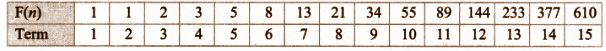(ii) F(8) = F(7) + F(6)

Given F (n) is a Fibonacci number and n = 8.

F(8) = F(7) + F(6) is any number in the Fibonacci series is the sum of preceding 2 terms.

(iii) Every 3rd number of the Fibonacci sequence is a multiple of 2.

(iv) Every 6th number of the Fibonacci sequence is a multiple of 8.

(v) 987

F(18) = F(17) + F(16)

F(18) – F(17) = F(16) = F(15) + F(14) = 610 + 377 = 987

Question 2: In the given graph sheet draw and colour how the Fibonacci number pattern makes a spiral in the Golden Rectangle.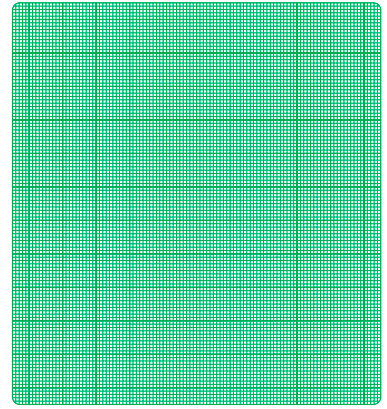Solution: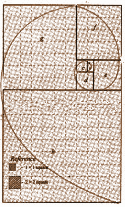### Samacheer Kalvi 8th Maths Term 2 Chapter 4: Information Processing Book Exercise 4.2 Questions and Solutions

Question 1: Write the common prime factors of 30 and 250.

Solution:

Prime factors of 30 are 2 * 3 * 5

Prime factors of 250 are 5 * 5 * 5 * 2

Common prime factors of 30 and 250 are 2 * 5 = 10

Question 2: Using the repeated division method and find HCF of 392 and 256.

Solution: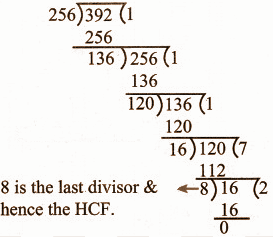HCF = 8

Question 3: Kalai wants to cut identical squares as big as she can, from a piece of paper measuring 168mm and by 196mm. What is the length of the side of the biggest square? (To find HCF using repeated subtraction method).

Solution:

m = 196 and n = 168

m – n = 196 – 168 = 28. Now, n = 28, m = 168

m – n = 168 – 28 = 140. Now, n = 28, m = 140

m – n = 140 – 28 = 112. Now, n = 28, m = 112

m – n = 112 – 28 = 84. Now, n = 28, m = 84

m – n = 84 – 28 = 56. Now, n = 28, m = 56

m – n = 56 – 28 = 28.

HCF = 28

Length of the side of the biggest square is 28mm.

### Samacheer Kalvi 8th Maths Term 2 Chapter 4: Information Processing Book Exercise 4.3 Questions and Solutions

Question 1: Frame Additive cipher table (key = 4).

Solution: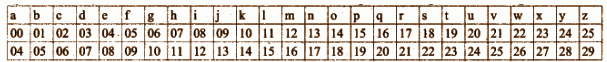Question 2: A message like “Good Morning” written in reverse would instead be “Doog Gninrom”. In the same way decode the sentence given below: “Ot dnatsrednu taht scitamehtam nac eb decneirepxe erehwreve ni erutan dna laer efil.”

Solution:

“To understand that mathematics can be experienced wherever in nature and real-life.”

Question 3: Decode the given Pigpen Cipher text.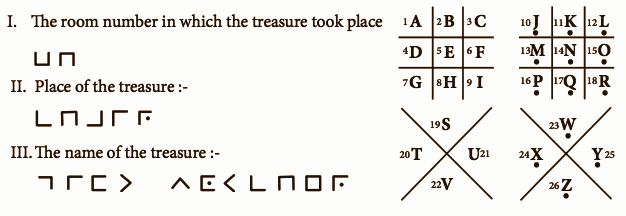Solution:

(i) The room in which the treasure took place = 28.

(ii) The place of treasure is the chair.

(iii) Identity of treasure is the gift voucher.

### Samacheer Kalvi 8th Maths Term 2 Chapter 4: Information Processing Book Exercise 4.4 Questions and Solutions

Question 1: The rule of Fibonacci Sequence is F(n) = F(n – 2) + F(n – 1). Find the 11th to 20th Fibonacci numbers.

Solution:

Fibonacci series is given by F(n) = F(n – 2) + F(n – 1)

F(11) = F(11 – 2) + F(11 – 1) = F(9) + F(10)

F(12) = F(12 – 2) + F(12 – 1) = F(10) + F(11)

………….

F(20) = F(19) + F(18)

The Fibonacci series is given by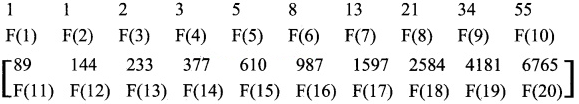Question 2: Praveen recently got the registration number for his new two-wheeler. Here, the number is given in the form of mirror-image. Encode the image and find the correct registration number of Praveen’s two-wheeler.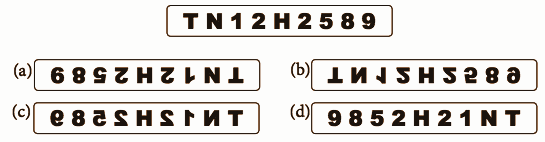Solution:

The mirror image is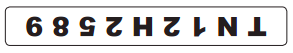So, the correct option is C.